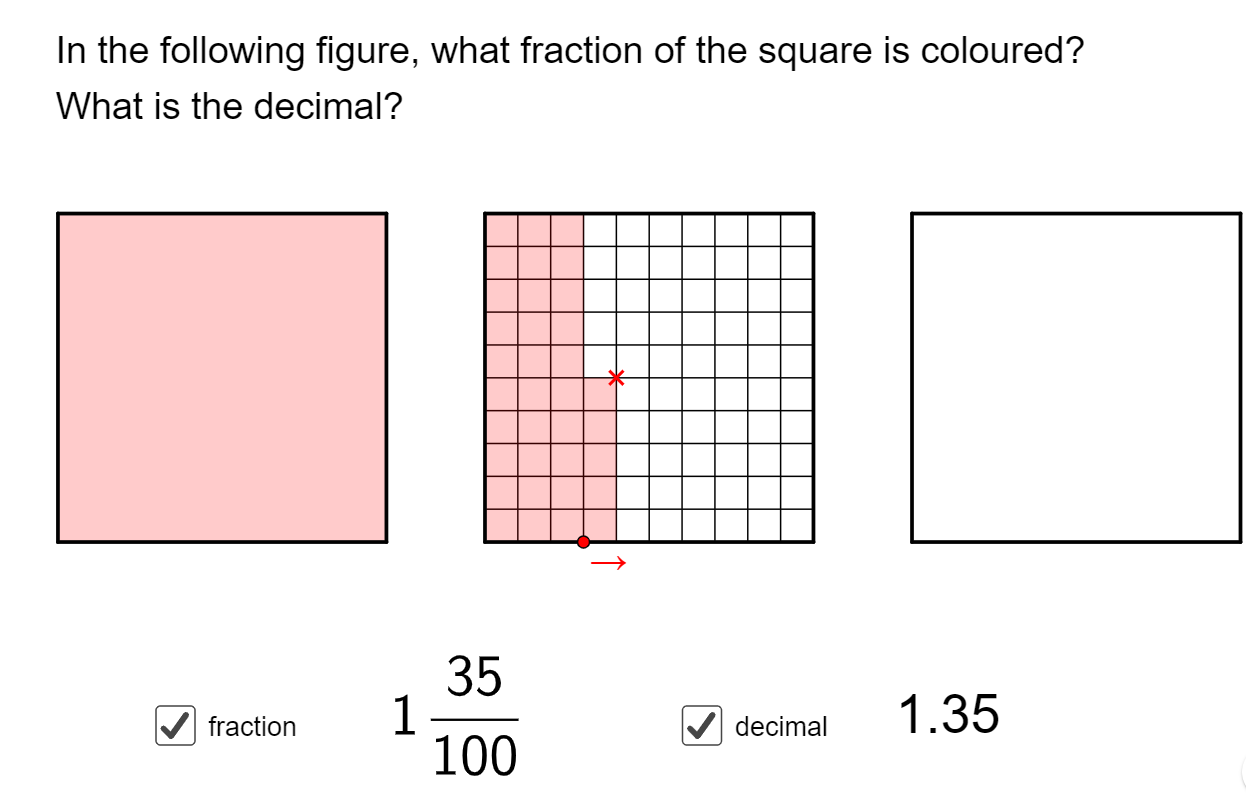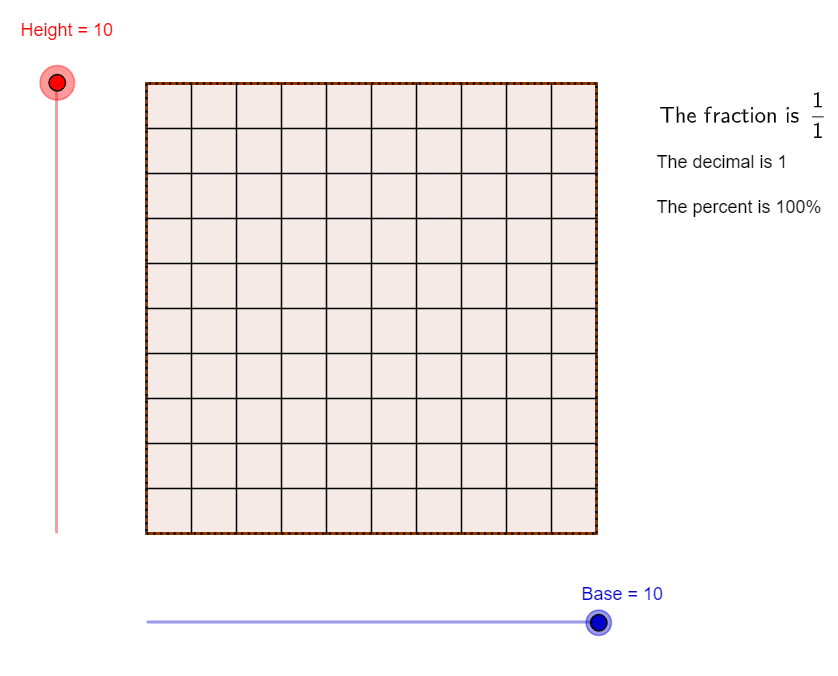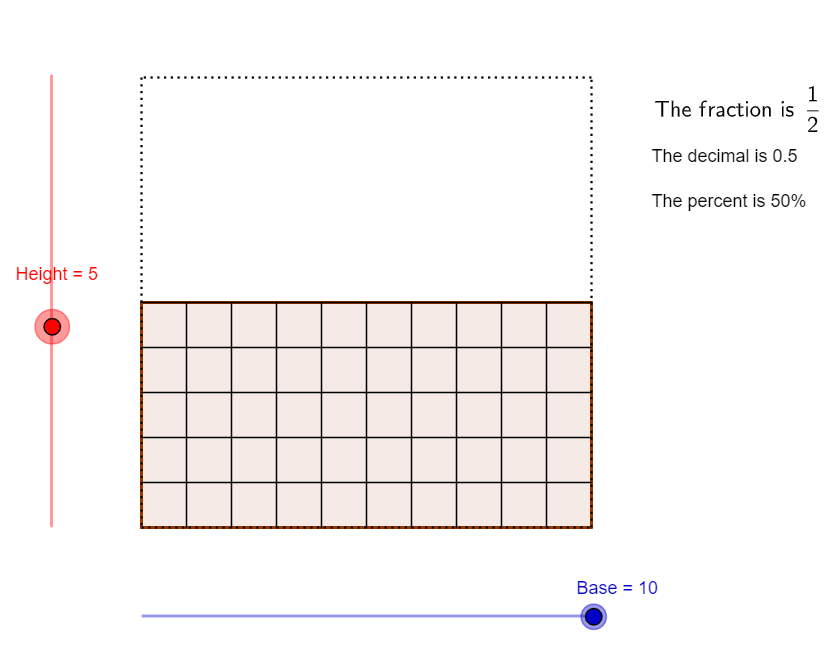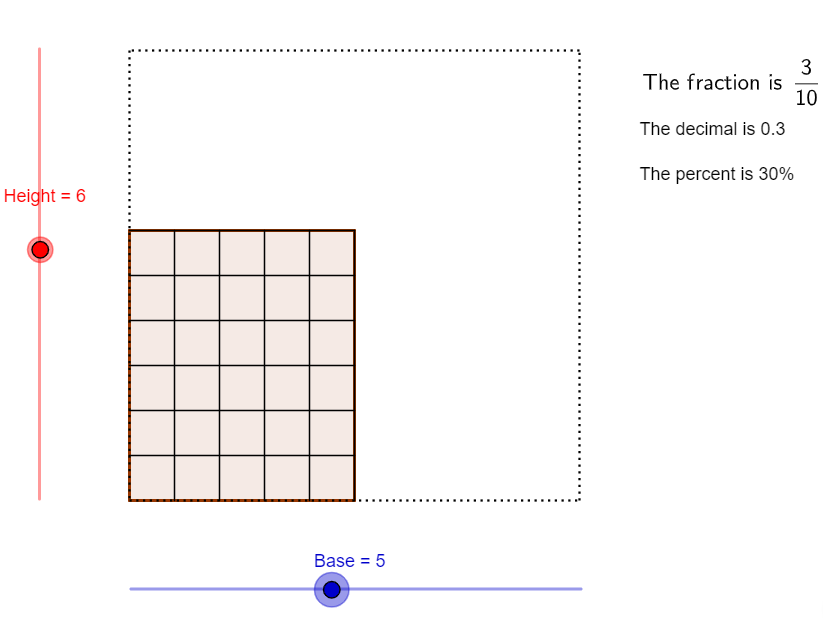# Relationship between Fractions and Decimals

Go back to  'Decimals'

## Introduction to Relationship Between Fractions and Decimals

Often fractions and decimals are presented as two unrelated topics. This means children don’t use their knowledge of fractions to understand decimals. Instead they try and memorise all procedures and formulae independently leading to frustration, confusion and poor conceptual clarity. Well, we’re here to show you that these two entities are completely interconvertible!

## The Big Idea: What governs this relationship between fractions and decimals ?

The fascinating concept behind this relationship is that EVERY decimal can be written as a fraction by dividing the number with the number of decimal places it holds.

### Converting fractions to decimals

Given the way decimals are defined, there is a natural connection between them and fractions. Our place value system allows us to have place names less than ones. One-tenth is the first digit to the right of the decimal point. One-hundredth is the second place to the right after the decimal point. These naturally refer to the fractions \begin{align}\frac{1}{{10}}\end{align} and \begin{align}\frac{1}{{100}}\end{align}.

Let us say you have 5 apples, of which you gave away 2. The fraction of your stash which you now have left is \begin{align}\frac{3}{{5}}\end{align}. If I ask you to represent this in decimal form, all you have to do is look at both numerator and denominator along with their periods or decimal points. So;

\begin{align} & {\frac{3}{5} = \frac{{{\rm{ }}3.0}}{5}}\\ & { = \frac{{30}}{{\left( {5 \times 10} \right)}}}\\ & { = \frac{{30}}{5} \times \frac{1}{{10}}}\\ & = 6 \times \frac{1}{{10}}\\ & = 6 \times 0.1\\ & = 0.6 \end{align}

Have a go yourself, take a look at the visual representation of this.### Converting decimals to fractions

What happens if you get the opposite problem where you have a decimal which you need to convert to a fraction? This is where you apply the base ten. Let us say you have a decimal 0.25 which you need to convert to a fraction. You need to write the decimal to the correct base and then consider as denominator the base of ten which the number has. Once you get the fraction, just check if it can be further simplified. Let us see how this can be done:

\begin{align} & 0.25 \\ & =\frac{0.25}{1} \\ & =\frac{\left( 0.25\times 100 \right)}{\left( 1\times 100 \right)} \\ & =\frac{25}{100} \\ & =\frac{1}{4} \\ \end{align}

### Converting a decimal to a percentage

Every decimal is built on the base of ten, as we said earlier. And a percentage is nothing, but a fraction expressed in terms of the number 100 as a denominator. So, how do we look at a decimal as a percentage? Let us look at the same decimal 0.25 and see how we can convert it to a percentage. The aim would be to have a fraction with 100 as the denominator.

So;

\begin{align}& 0.25 \\& =\frac{25}{100} \\ & \text{ = 25%} \\ \end{align}

Let us look at another decimal which doesn’t have 100 as the denominator automatically, like

\begin{align} & {0.027}\\ & { = \frac{{0.027}}{1}}\\ & { = \frac{{27}}{{1000}}}\\ & { = \frac{{2.7}}{{100}}}\\ & \text{= 2.7 %} \end{align}

Take a look here to understand the relation between fractions, decimals, and percentages. These three images represent 3 different cases to help ensure that you can visualize the nature of this relationship.## How is it important?

### Look left, look right

In later chapters we will look at how to add, subtract, multiply and divide decimals. But let us end this session by telling you about the easy way to multiply or divide decimals with any number which is 10 or its multiple. The trick here is that the decimal point just needs to be moved certain places to the right or to the left, depending whether you are multiplying or dividing respectively. The number of places to be moved depends on the multiple of 10 used.

\begin{align} &{\rm{0}}{\rm{.235 \times 100}}\;{\rm{ = }}\;{\rm{23}}{\rm{.5}} \\ & \left( {{\text{100 has 2 zeroes, move the decimal point 2 places to the right}}} \right)\\\\ &{\rm{0}}{\rm{.235 \times 10000}}\;{\rm{ = }}\;{\rm{2350}}\\ & \left( {{\text{10000 has 4 zeroes, move the decimal point 4 places to the right}}} \right)\\\\ &\frac{{{\rm{0}}{\rm{.235}}}}{{{\rm{10}}}}\;{\rm{ = }}\;{\rm{0}}{\rm{.0235}}\\ & \left( {{\text{10 has 1 zero, move the decimal point 1 place to the left}}} \right)\\\\ &\frac{{{\rm{0}}{\rm{.235}}}}{{1000}}\;{\rm{ = }}\;{\rm{0}}{\rm{.000235}}\\ & \left( {{\text{1000 has 3 zeroes, move the decimal point 3 places to the left}}} \right) \end{align}

## Common mistakes or misconceptions

• Children see whole numbers, fractions and decimals as three different and independent set of numbers. This means they will unable to compare a fraction with a decimal. E.g. won't be able to say which is bigger: $$\frac{3}{4}$$ or $$0.65$$.

• Children read numbers incorrectly. E.g. $$1.23$$ is read as “One point twenty three” instead of “one point two three”.

This happens because students have not fully internalised the place values lower than one.

## Tips and Tricks

• Get students to plot a few whole numbers, fractions and decimals all on the same number line. Let them see that while fractions and decimals look different, they are all numbers that can be compared with each other.